### Contravariance and Covariance - Part 3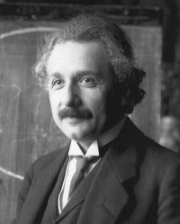Albert Einstein
Tensor Calculus

Vector Concept 3 -- Classical Tensor Analysis in Rn
Our transformations of coordinates have hitherto mostly involved constants in the change of coordinates transformation matrix. We now extend the allowed transformations to include more general functions, from which we get yet another definition of a vector.
It will turn out, for general non-cartesian coordinates, there are two possible transformation laws. One law describes contravariant vectors, and the other covariant vectors.
While part 2 required some knowledge of algebra, this part will require some knowledge of multi-variable calculus. For brevity, I give only the essential concepts. As a minimum, some knowledge of the chain rule would be needed to follow along.
Curvilinear Coordinates

Let x1,...,xn be a rectangular coordinate system in Rn. (It should perhaps be remarked that it in general mathematical spaces, it is not always possible to find such a coordinate sytem. The existence of a global rectangular coordinate system is equivalent to assuming the space is Euclidean.) Consider the general transformation of coordinates to a new, not necessarily rectangular, coordinate system y1,...,yn:
 y1 = y1(x1,...,xn) ...      ............... ...      ............... ...      ............... yn = yn(x1,...,xn) (Eq. 1)

We require certain technical conditions in order that our new coordinate system be well defined. In particular, the transformation should be differentiable and have a differentiable inverse. Sufficient conditions for this are given by the implicit function theorem -- the Jacobian of the transformation should be non-zero. The y-coordinate system so defined constitutes a curvilinear coordinate system.
Now that curvilinear coordinates have been introduced, we can consider transformations from one curvilinear coordinate system to a different curvilinear coordinate system... which brings us to the classical idea of a tensor and the corresponding definitions:
##### Scalar
A function f(x1,...,xn) is a scalar if under a change of coordinates (1), it does not change value.

In other words, if f, or f prime, is the value in the new y-coordinate system (f prime is not the derivative!),
f(y1,...,yn) = f(x1,...,xn)
Typical examples of scalars are mass and temperature, which are independent of choice of coordinates. A scalar is also called a tensor of rank 0 . Vectors, which we now define, are tensors of rank 1.
##### Contravariant Vector
A contravariant vector is a set of functions vk  that, under a change of coordinates, transform as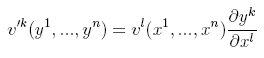(Eq. 2)
where the Einstein summation convention has been used to indicate a summation over the repeated index l

##### Covariant Vector
A covariant vector is a set of functions vk   that, under a change of coordinates, transform as(Eq. 3)
where the Einstein summation convention has been used to indicate a summation over the repeated index l.
The origin of the above definitions may not at first be obvious. However, if we expand the standard position "vector" P(x1,...xn) in the standard rectangular coordinate basis {i1,...,in} ,the relation to our previous definitions becomes more apparent.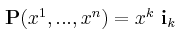(Eq. 4)
we see the rectangular basis "vectors", {i1,...,in},  are given by(Eq. 5)
Now, changing coordinates to y-coordinate system, we find(Eq. 6)
Examining this equation for ik, we recognize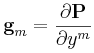(Eq. 7)
as the tangent vector to the ym coordinate curve. The set of vectors gm    are known as the curvilinear basis vectors for the y-coordinate system, and form a basis for Rn (That they form a basis is gauranteed by virtue of the fact that the Jacobian of the transformation is non-zero). Therefore, any vector v in Rn can be expanded as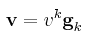(Eq. 8)
The vk are the contravariant components of v. Denoting the dual basis to the {gm} by {gm}, we can expand v in the dual basis as(Eq. 9)

The vk are the covariant components of v.
It might come as a surprise that in the most general case in this definition, when non-orthognal transformations are allowed, a finite directed line segment will not in general obey the transformation laws (2) or (3) and so is NOT a vector. However, infinitesimal directed line segments are vectors.

This whole analysis has assumed the existence of a global rectangular coordinate system, or in other words, that the space we are considering is Euclidean. Easing this restriction, and considering non-Euclidean spaces, leads us to the study of vectors defined on more general spaces called manifolds.

Next week -- Vectors on Manifolds.